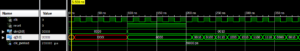# VHDL Code for 4-Bit Aynchronous Accumulator

### Accumulator

Accumulator work similar to the functionality of counter. The main difference is instead increment the counter value by constant, Accumulator add the input value with the current value.

### Accumulator Block Diagram### VHDL Code for 4-bit Asynchronous Accumulator

```library ieee;
use ieee.std_logic_1164.all;
use ieee.std_logic_unsigned.all;

entity accumulator is
port(clk, reset : in std_logic;
Din : in std_logic_vector(3 downto 0);
Q : out std_logic_vector(3 downto 0));
end accumulator;
architecture bhv of accumulator is
signal tmp: std_logic_vector(3 downto 0);
begin
process (clk, reset)
begin
if (reset='1') then
tmp <= "0000";
elsif rising_edge(clk) then
tmp <= tmp + Din;
end if;
end process;
Q <= tmp;
end bhv;```

### TestBench VHDL Code for 4-bit Asynchronous Accumulator

```LIBRARY ieee;
USE ieee.std_logic_1164.ALL;

-- Uncomment the following library declaration if using
-- arithmetic functions with Signed or Unsigned values
--USE ieee.numeric_std.ALL;

ENTITY tb_accumulator IS
END tb_accumulator;

ARCHITECTURE behavior OF tb_accumulator IS

-- Component Declaration for the Unit Under Test (UUT)

COMPONENT accumulator
PORT(
clk : IN std_logic;
reset : IN std_logic;
Din : IN std_logic_vector(3 downto 0);
Q : OUT std_logic_vector(3 downto 0)
);
END COMPONENT;

--Inputs
signal clk : std_logic := '0';
signal reset : std_logic := '0';
signal Din : std_logic_vector(3 downto 0) := (others => '0');

--Outputs
signal Q : std_logic_vector(3 downto 0);

-- Clock period definitions
constant clk_period : time := 20 ns;

BEGIN

-- Instantiate the Unit Under Test (UUT)
uut: accumulator PORT MAP (
clk => clk,
reset => reset,
Din => Din,
Q => Q
);

-- Clock process definitions
clk_process :process
begin
clk <= '0';
wait for clk_period/2;
clk <= '1';
wait for clk_period/2;
end process;

-- Stimulus process
stim_proc: process
begin
-- hold reset state for 100 ns.
wait for 100 ns;

reset <= '1';

Din <= "0010";

wait for 100 ns;

reset <= '0';

wait;

end process;

END;```

### Output Waveform for Accumulator VHDL Code×

Chat with us for queries on the EDGE FPGA kit

×